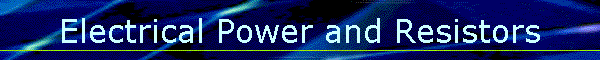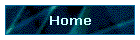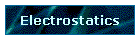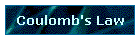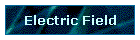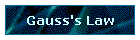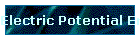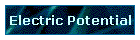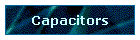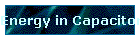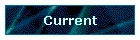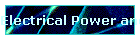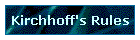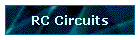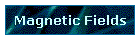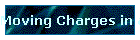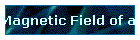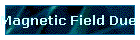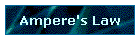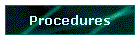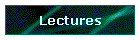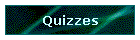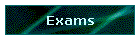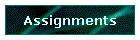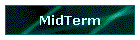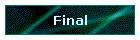Phys 4, Section 2                                                                 Feb 6, 2002   Power dissipation Current flow through a resistor causes heating (called Joule heating or resistive heating).  This represents a loss of energy from the system Power is rate of doing work, so for electrical quantities, dW = Vdq P = dW/dt =Vdq/dt = VI = I2R = V2/R Resistor Combinations Parallel:    1/Reff = 1/R1 + 1/R2 + ... Series:    Reff = R1 + R2 + ... Effective resistance of resistors in series is always more than the largest resistor in the set, while the effective resistance of resistors in parallel is always less than the smallest resistor in the set.